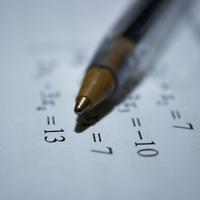# Rearranging equationsAlgebra is the process by which an equation can be manipulated to make another quantity the subject of the equation.

To access the contents of this site, you need to log in or subscribe